# Reservoir Simulation - Technical Description

The reservoir simulation module is designed to model depletion performance in tight unconventionals. The OPM Flow reservoir simulator, an open-source simulator using Eclipse 100 input syntax, is the engine of the tool.

## 1. Theory

### 1.1 Wellbox Model

The simulations are performed on a prebuilt model that represents the typical well in tight unconventionals, often referred to as a multi-fractured horizontal well (MFHW). The prebuilt model is called the wellbox model. A cartoon of the wellbox model is shown in Fig. 1.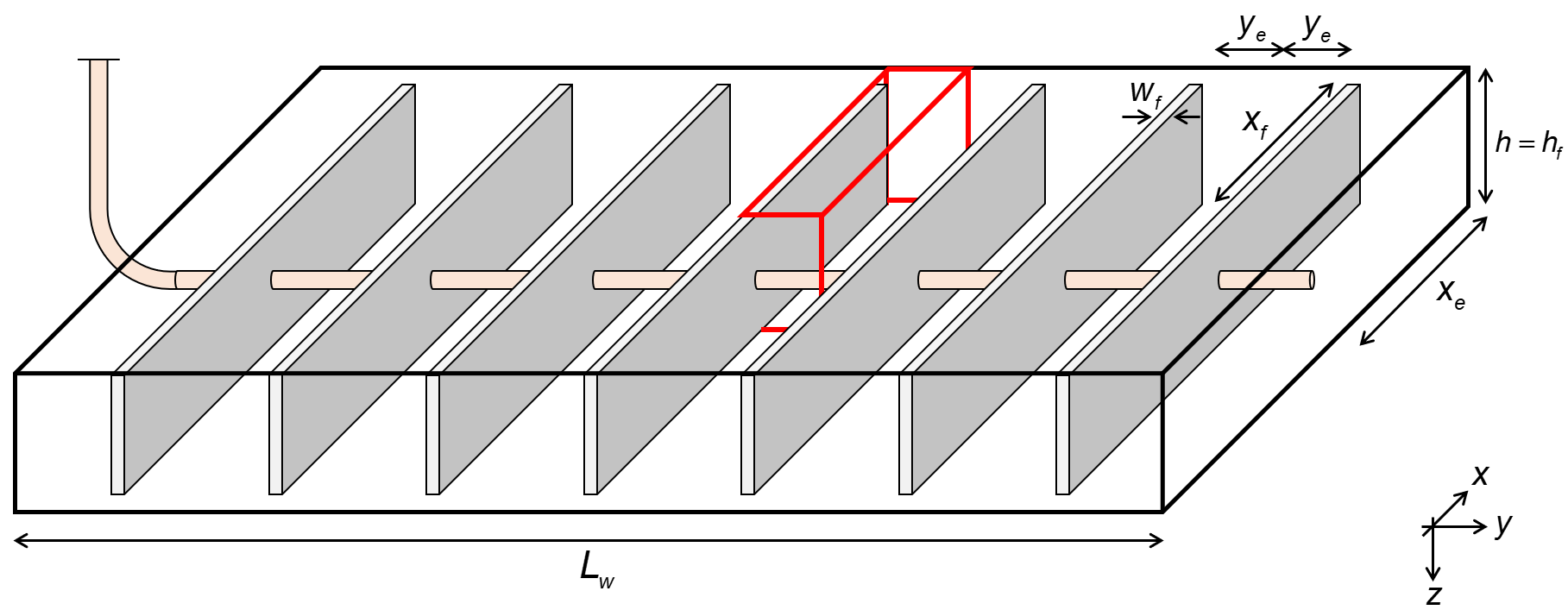Fig. 1: Wellbox model

The wellbox model will have dimensions that the user can change, but a set of default values will be given initially. The dimensions and default values are as follows:

Dimension Symbol Default Value
Lateral Length $$L_w$$ 5280 ft
Reservoir Half-Extent $$x_e$$ 800 ft
No. of Fractures $$N_f$$ 100
Fracture Half-Length $$x_f$$ 400 ft
Reservoir and Fracture Height $$h_f$$ 150 ft

As the wellbox model is symmetric, the numerical model will be a one-quarter fracture, as highlighted by a red-lined box in Fig. 1. The production from the numerical model is upscaled to by using a scaling factor. The scaling factor is defined as

where $$N_f$$ is the number of hydraulic fractures (clusters) in the wellbox model. The dimensions of the numerical model are the same as the wellbox model, except for the y-dimension, where instead of $$L_w$$, we use $$y_e$$ defined as

### 1.2 Enhanced Fracture Region (EFR) Option

It is possible to introduce a region of enhanced, effective permeability, $$k_{EFR}$$, around each fracture with extent, $$y_{EFR}$$. An example description for a single-fracture is illustrated in Fig. 2. The reservoir can extend beyond the fracture tip, and have contributions beyond the fracture height. The enhanced fracture region has the same height and length as the fracture, only difference being the extent in the y direction.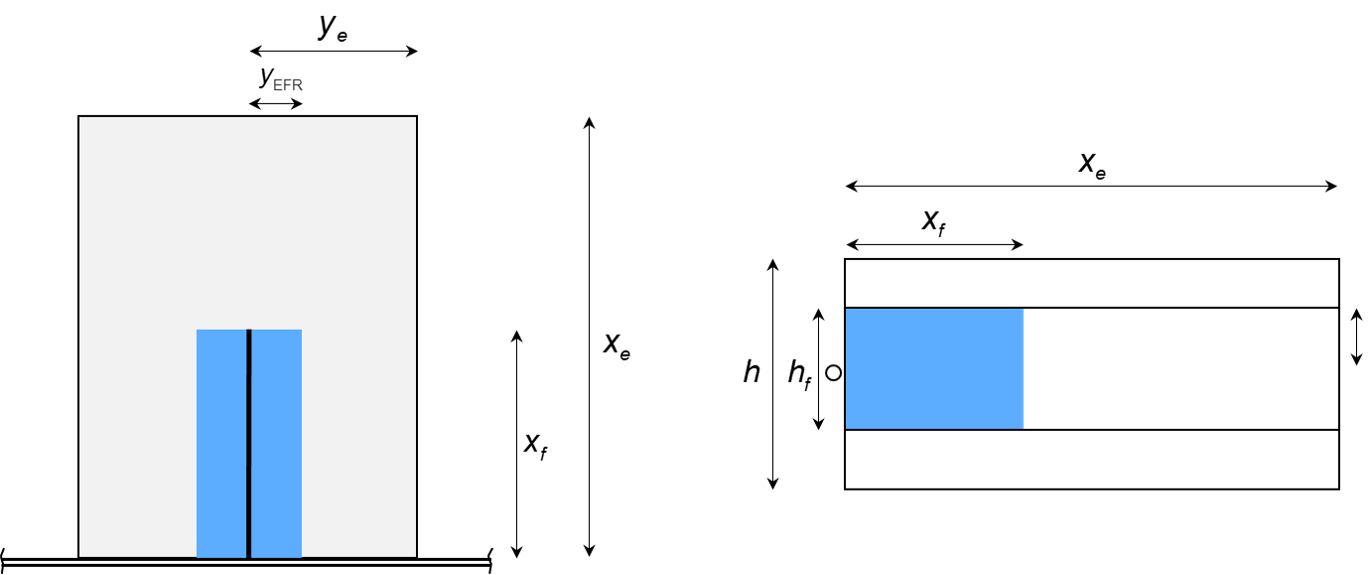Fig. 2: Enhanced fracture region model

### 1.3 Reservoir Properties

#### 1.3.1 Grid-Block Properties

The reservoir properties will include some properties that can be changed by the user, and some properties that are locked. The changeable reservoir properties are given the following table:

Property Symbol Default Value
Matrix Porosity $$\phi_m$$ 0.03
Matrix Permeability $$k_m$$ 100 nd
Matrix Initial HC Saturation (Fluid Dep.) $$S_{HCi}$$ 1
Matrix Initial Water Saturation $$S_{wi}$$ $$S_{wc}$$
Initial Reservoir Pressure $$p_{Ri}$$ 7500 psia
Hydraulic-Fracture Dimensionless Conductivity $$F_{CD}$$ 100

The locked reservoir properties are as follows

Property Symbol Default Value
Fracture Porosity $$\phi_f$$ 0.1
Fracture Width $$w_f$$ 0.1 ft
Depth $$D$$ 10000 ft

Note that depth is merely a cosmetic property as there is only one horizontal layer in the model, and no tubing calculations are made.

The dimensionless fracture conductivity is defined as

We calculate $$k_f$$ from equation (3).

#### 1.3.2 Saturation-Dependent Properties

##### 1.3.2.1 Two-Phase Relative Permeability

The saturation-dependent properties in this model are the relative-permeability curves. We use the Corey-type model to express two-phase relative permeability. Baker's linear interpolation model is used to calculate $$k_{ro} = f(S_w,S_g)$$ in three-phase systems.

The Corey-type model calculates $$k_{rw} = f(S_w), k_{row} = f(S_w)$$, $$k_{rog} = f(S_g)$$, and $$k_{rg} = f(S_g)$$ as follows:

where the following parameters must be provided

Property Symbol Default Value Matrix Default Value Fracture
Connate Water Saturation $$S_{wc}$$ 0.2 0
Residual Oil Saturation (O-W) $$S_{orw}$$ 0.2 0
Residual Oil Saturation (O-G) $$S_{org}$$ 0.2 0
Critical Gas Saturation $$S_{gc}$$ 0.05 0
Water Corey Exponent $$n_w$$ 2 1
Oil Corey Exponent (O-W) $$n_{ow}$$ 2 1
Oil Corey Exponent (O-G) $$n_{og}$$ 2 1
Gas Corey Exponent $$n_g$$ 2 1
Water Relative Permeability at $$S_{orw}$$ $$k_{rwro}$$ 1 1
Oil Relative Permeability at $$S_{wc}$$ $$k_{rocw}$$ 1 1
Gas Relative Permeability at $$S_{org}$$ $$k_{rgro}$$ 1 1

The relative-permeability values in the matrix will be open for change by the user. The fracture values are locked.

##### 1.3.2.2 Three-Phase Relative Permeability

To achieve platform consistency between the different simulators (OPM Flow, Eclipse 100, Sensor, CMG IMEX), we have found that the Baker linear interpolation of $$k_{ro}$$ is the only three-phase relative permeability that is common for all simulators.

The Baker linear interpolation correlation is as follows

The testing of OPM Flow has been done by comparing OPM Flow to Sensor. The default three-phase relative permeability correlation in Sensor is Stone 1 with Fayer's and Matthew's expression for $$S_{om}$$. This option is not available in OPM Flow, which results in inconsistencies in predicted results for the two simulators. The default relative permeability correlation for OPM Flow (and E100) is the Baker linear interpolation. This correlation can also be activated in Sensor, making the two simulators consistent. However, Stone 1 with Fayer's and Matthew's expression for $$S_{om}$$ remains the preferred choice, which was also the case for Keith Coats (see quote below)

The default three-phase relative-permeability correlation for CMG IMEX is Stone 2. Hence, for consistency, our output files to CMG IMEX format will contain a keyword activating the Baker linear interpolation correlation.

The table below summarizes the three-phase correlations available in the different simulators.

Three-Phase Rel.Perm. Correlation Sensor OPM FLow Eclipse 100 CMG IMEX
Stone 1 X X X
Stone 1 with Fayer's and Matthew's X X X
Stone 2 X X X X
Baker X X X X

Quote

"I would never use Baker kr. Stone 2 kr is pathological and Baker kr may be. To my knowledge Stone 1 – Fayers kr (sensor default) is the only kr logic free of pathology"

"Default kro is Stone 1 with Fayer’s modification. The ID option STONE 2 can be pathological, depending upon entered water-oil and gas-oil krow and krog relperm data. The pathology is a step-function change from kro>0 to kro=0 for an epsilon change in saturations. The default Stone 1 is not pathological"

*Default refers to Sensor

Keith Coats

#### 1.3.3 Multi-Layer Models

In whitson+, it is possible to have more than single layer in the model with fully or partially penetrating fracture.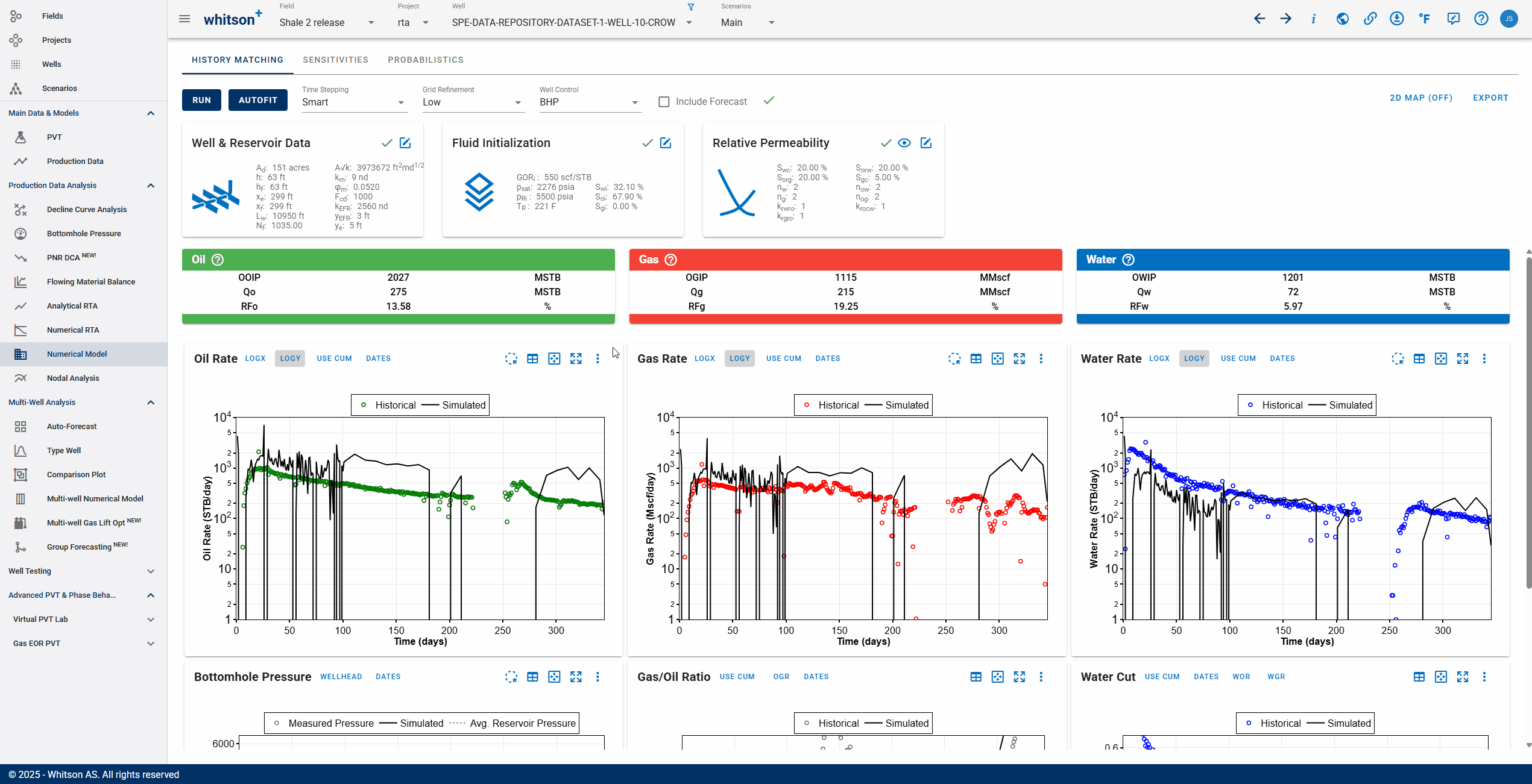The fractured layer properties are calculated by height weighted average of the $$n$$ penetrated layers, as follows

### 1.4 Fluid Properties

whitsonʳˢ is designed to do simple and fast reservoir modeling to evaluate depletion performance. To make this tool as efficient as possible, the modified black-oil PVT model (outlined below) is used for the fluid treatment. An important feature of whitsonʳˢ is the direct link to whitsonᴾⱽᵀ. This makes the generation of black-oil PVT tables a backend feature in whitsonʳˢ, where the user only needs to provide key properties related to fluid PVT such as temperature, surface-process information (separator pressure and temperature), and initial gas/oil ratio.

The key parameters of the modified black-oil PVT model are (details and definitions are found in Whitson and Brulé 2000) as follows:

Property Symbol
Oil Formation Volume Factor $$B_{o}$$
Solution Gas/Oil Ratio $$R_s$$
Dry-Gas Formation Volume Factor $$B_{gd}$$
Solution Oil/Gas Ratio $$r_s$$
Oil Viscosity $$\mu_o$$
Gas Viscosity $$\mu_g$$

whitsonʳˢ will return the in-place volumes of the wellbox model when all model dimensions, reservoir properties, and fluid properties have been specified. The in-place numbers are calculated as

### 1.5 Water and Rock Properties

The following water and rock properties are used as default values for all reservoir simulators.

Property Symbol Default Value
Water Formation Volume Factor at $$p_{ref}$$ & $$T_R$$ $$B_w$$ 1 RB/STB
Water compressibility $$c_w$$ 3x10$$^{-6}$$ 1/psia
Surface water density $$\rho_w$$ 62.37 lb/ft$$^3$$
Water viscosity $$\mu_w$$ 0.5 cp
Reference pressure for $$B_w$$ $$p_{ref}$$ 14.696 psia
Formation (rock) compressibility $$c_r$$ 4x10$$^{-6}$$ 1/psia

### 1.6 Geomechanical Effects - Pressure-Dependent Permeability

The matrix, EFR (if activated), and hydraulic fracture can be assigned a pressure-dependent permeability on the form where $$\gamma$$ is a property that be assigned in the frontend. There are two different gamma values, on for the hydraulic fracture $$\gamma_f$$, and one for the matrix and EFR (if activated) $$\gamma_r$$. To activate the pressure-dependent permeability, toggle on "Pressure-Dependent Permeability" under the "Well & Reservoir Data" card in the "History Matching" module.

### 1.7 Computing Original Gas in Place with Adsorption

Ambrose et al. argue that the free OGIP must be corrected for the adsorbed gas that occupies some of the hydrocarbon pore volume (HCPV). If porosity is estimated from core plugs where core preparation has removed the adsorbed gas, then the OGIP will be overestimated.

The convention of reporting OGIP when adsorption is included, is to report it in units of scf/ton (standard cubic feet per short ton). For a fluid system having only dry/wet gas and water, the total OGIP is

where

and with $$C_1\approx 5.7060$$ and $$C_2\approx1.318\cdot10^{-6}$$ are unit conversion factors.

The OGIP resolved in GFMB is the free OGIP. We can use this volume to compute the rock mass, which in turn can be used to calculate the adsorbed OGIP. Multiplying equation \eqref{eq:freegasperton} with the rock mass ($$G=\hat{G}_f m_r$$) gives the free OGIP in units of scf. Solving for the rock mass yields

This rock mass can then be multiplied by equation \eqref{eq:adsorbedgasperton} to get the adsorbed OGIP in scf, i.e.,

## 2. Numerical Model

### 2.1 Gridding

The numerical model will have a gridding as shown in Fig. 3. The gridding is logarithmic in the y-direction, and in the x-direction beyond the fracture tip. From the wellbore to the fracture tip the gridding is uniform in the x-direction.Fig. 3: Gridding of Numerical Model

The gridding is calculated based on the dimensions of the model. The gridding of the numerical model will is a backend feature, where the user can choose between low, medium, and high grid refinement. Determining the number of grid blocks for the chosen level of grid refinement is discussed in the next subsection. The formulas used for gridding are

where the total number of grid blocks in the model are $$N_t = (N_{x,i} + N_{x,o})N_y = N_xN_y$$.

### 2.2 Grid Refinement

The number of grid blocks in the model is controlled by the grid-refinement level set by the user. There are four levels of grid refinement which allow the user to decide the level of "compromise" between simulation run time and level of detail captured in the simulation results:

1. Very Low (favours short simulation time)
2. Low
3. Medium
4. High (favours capturing the details)

An internal logic is used to translate the level of grid refinement to a number of grid blocks. We use average grid-block dimensions in the $$x$$- and $$y$$- directions to set the refinement of the gridding. The following table contains the fixed numbers for $$\bar{\Delta} x$$ and $$\bar{\Delta} y$$ at the three grid-refinement levels.

Property $$\bar{\Delta} x$$ (ft) $$\bar{\Delta} y$$ (ft)
Very Low 200 8
Low 50 2
Medium 25 1
High 12.5 0.5

The number of grid blocks in the $$x$$- and $$y$$- directions are then calculated as

The split of grid blocks in the $$x$$-direction $$N_{x,i}/N_x$$ is linear with $$x_f/x_e$$. Because the number of grid blocks have to be integers, we round the numbers as follows:

The number of grid blocks outside the fracture tip are then calculated by $$N_{x,o} = N_x - N_{x,i}$$.

## 3. What affects producing GOR profile and how?

### 3.1 Reservoir Parameters

We observe a wide-range of producing GOR profiles in tight unconventionals. But which reservoir parameters change producing GOR when the bottomhole pressure is below the saturation pressure? And how?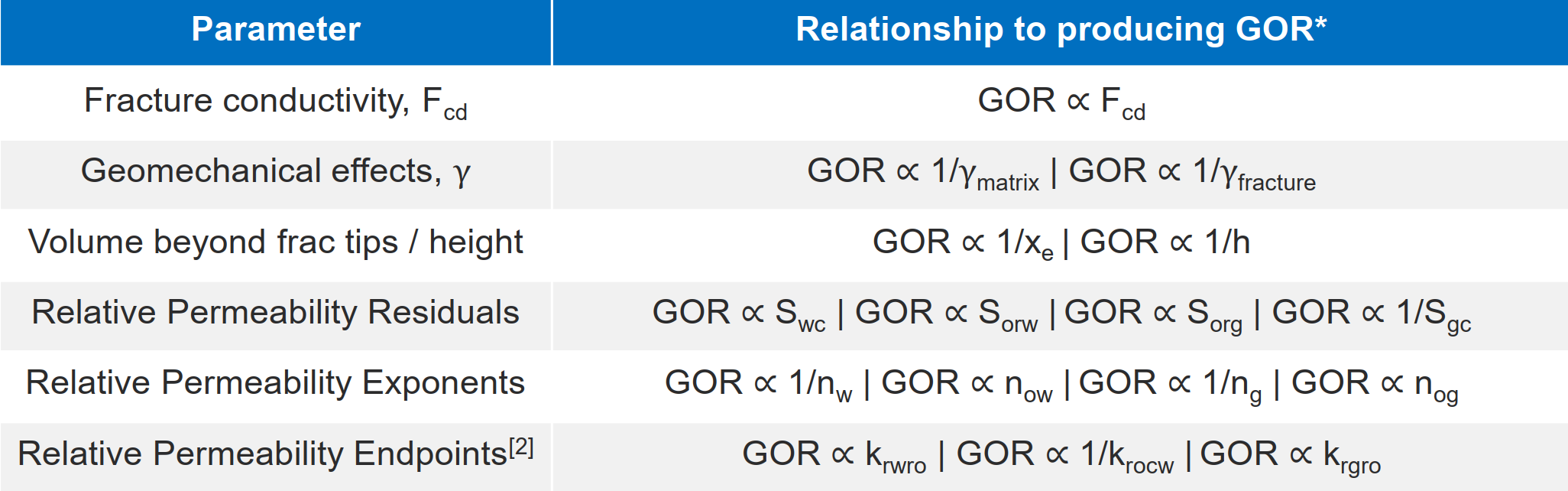Fig. 4: Effects of Reservoir Parameters on producing GOR

There are four broad categories of reservoir parameters that impact producing GOR

• Fracture conductivity
• Geomechanical effect
• Contributing volume beyond frac tips / height ("unstimulated rock")
• Relative permeability

This is summarized in the table above. Read more in this slide deck →→here←←.

### 3.2 Flow Regime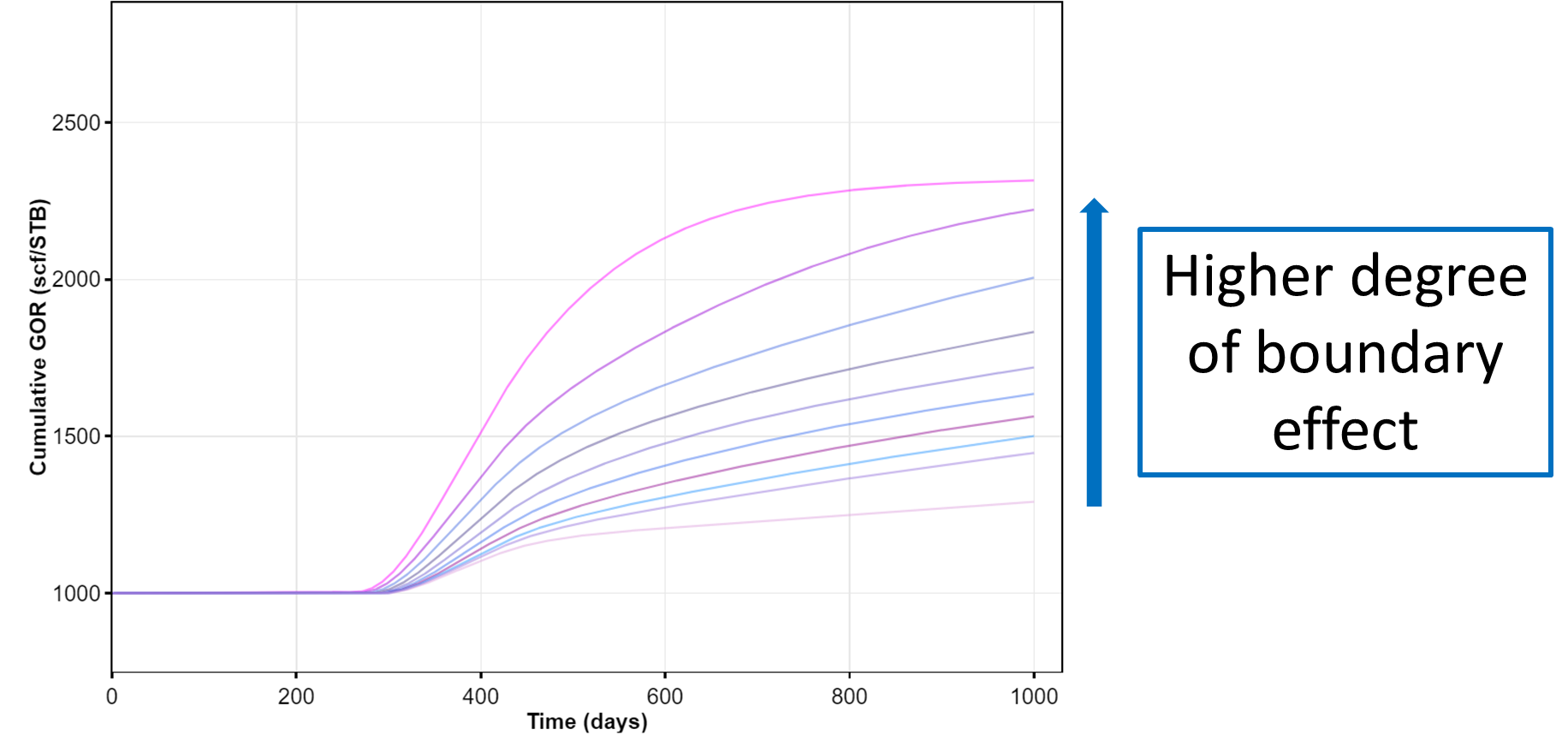Fig. 5: Effects of flow regime and boundaries on producing GOR profile.

In addition to the reservoir parameters outlined above, flow regime matters. Everything else being equal producing GOR follows this relationship below saturation pressure:

Where IA refers to infinite acting, TF refers to transitional flow and BDF refers to boundary dominated flow.

## 4. Forecast

### 4.1 BHP Forecast Type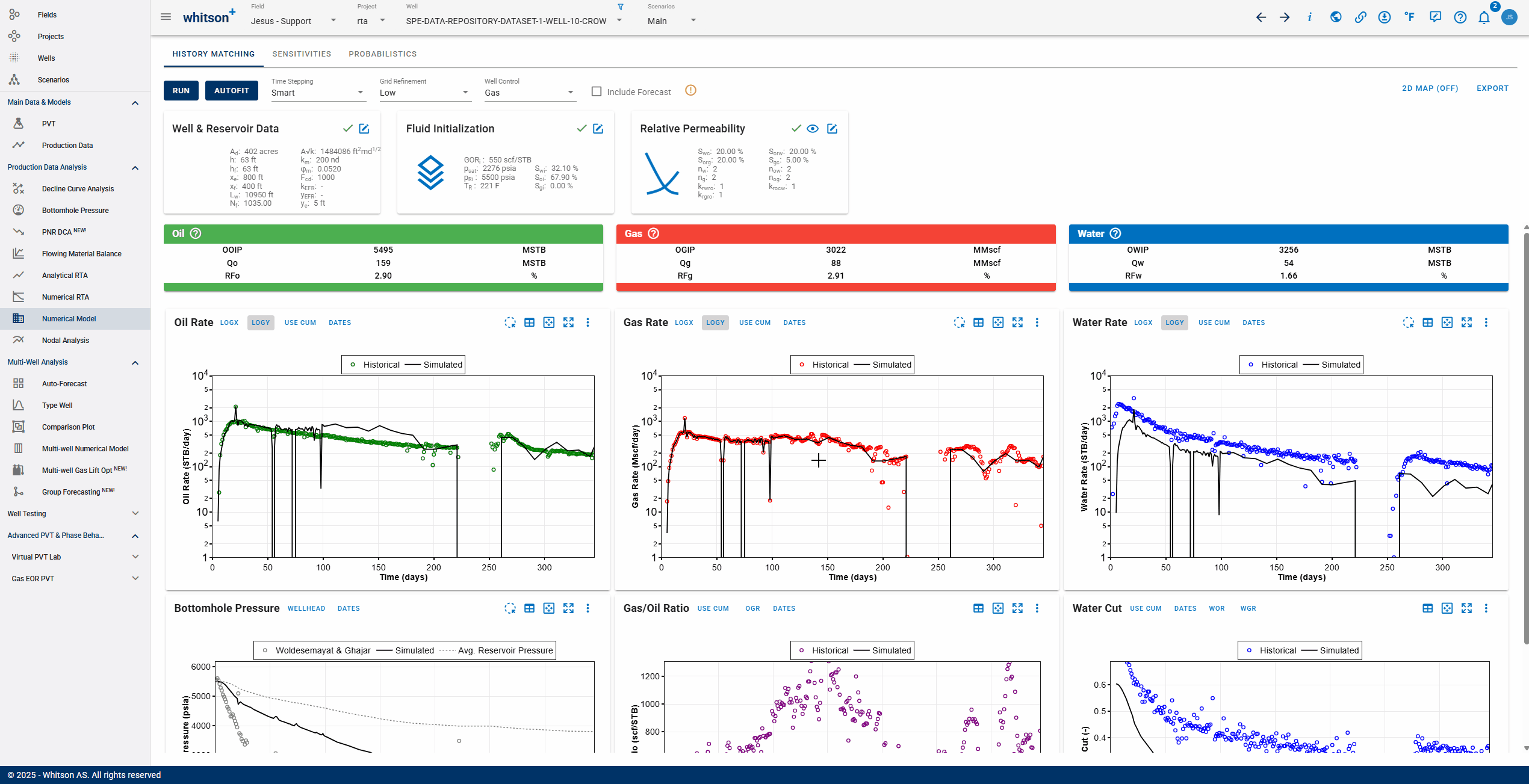Fig. 6: Forecasting the numerical model

After one has finished matching the production history, the results can be used to forecast the production. In whitson+, the simulation forecast is controlled by pressure (i.e. BHP or WHP). Whitson+ provides two alternatives in creating BHP forecast:

1. Constant BHP throughout simulation time.

2. Parametric Decline to set the forecasting function between the input initial and final BHP as hyperbolic or linear based on the input Nominal Pressure Decline in %/yr or psia/d, respectively.

3. Custom BHP provided by user in table-wise manner. By default, the first row will be the BHP at the last time step of history matching simulation run.

Forecasting Using WHP

More on WHP forecasting coming in this section soon!

## 5. Refrac Analysis -

A ‘refrac’ or refracturing treatment refers to the process of performing another hydraulic fracturing operation on a well that has already undergone the process in the past.

Typically, you may want to evaluate a refrac that was recently performed on one of the wells. To do that, use the MFMB feature - The workflow is outlined in this section here.

Sometimes, you also want to be able to predict the initial rates and subsequent decline for a refrac that is planned on a well in the future. To do that, use numerical modeling as shown below.

### 5.1 Workflow for estimating uplift on planned refracs

This section outlines our recommendation for what the simplified workflow could be -

1. History match the target well and resolve a numerical model with high confidence.
Then forecast this model to generate a baseline future expectation from the well, under current conditions as shown above in section 4 above.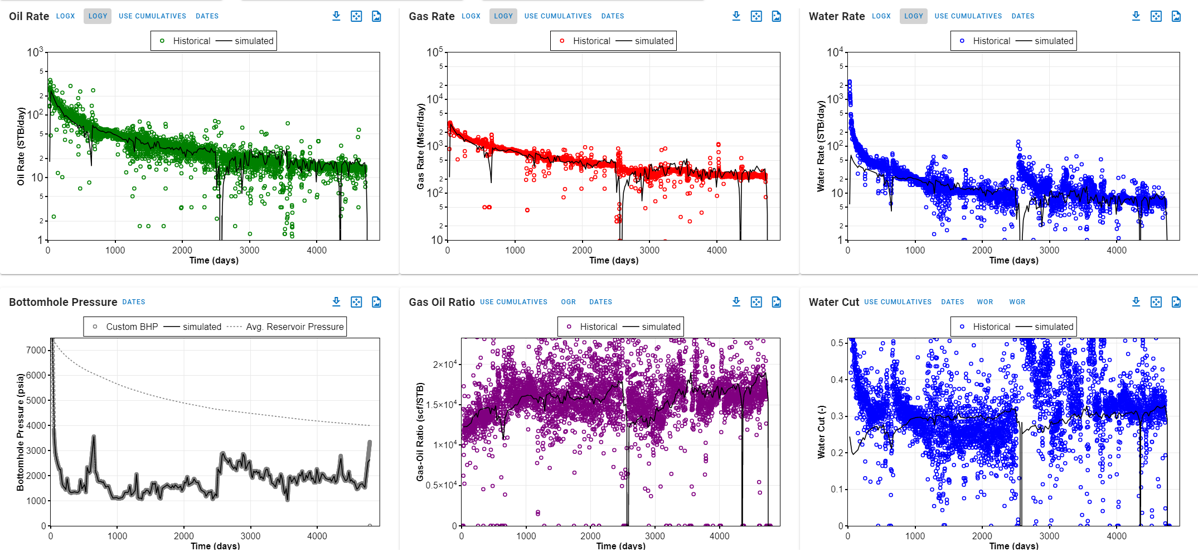Fig. 7: High confidence history matched numerical model

1. Copy the well (Wells page --> Click the three dots to the right of the well name --> Copy well)

2. On the copy of the well, modify the numerical model to reflect the expectation from the refrac, i.e. -
a. Change the completion - increase the number of fractures (Nf) or fracture half-length (xf) or both.
b. Increasing Nf will primarily accelerate depletion but yeild a higher intial rate.
c. Increasing xf (if we expect a bigger refrac completion) will add additional pore volume
d. Forecast this updated model.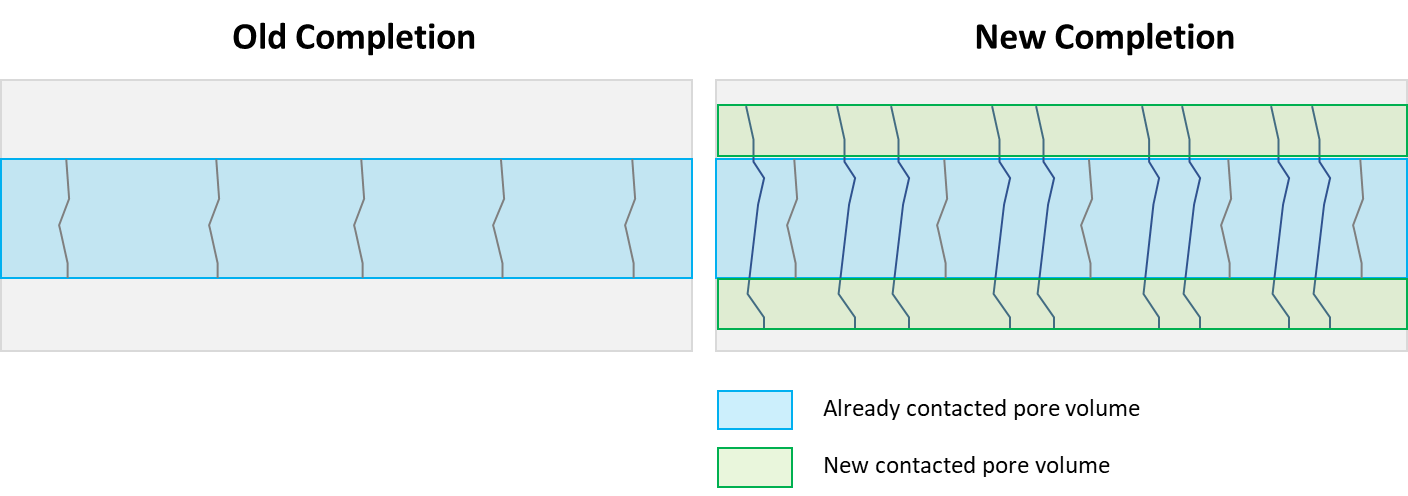Fig. 8: Updating the baseline numerical model

1. Delta between outcomes of Steps 1 and 2 is the refrac potential of this well.

2. Superpose these resulting rates at the time of refrac to get the uplift expected after refrac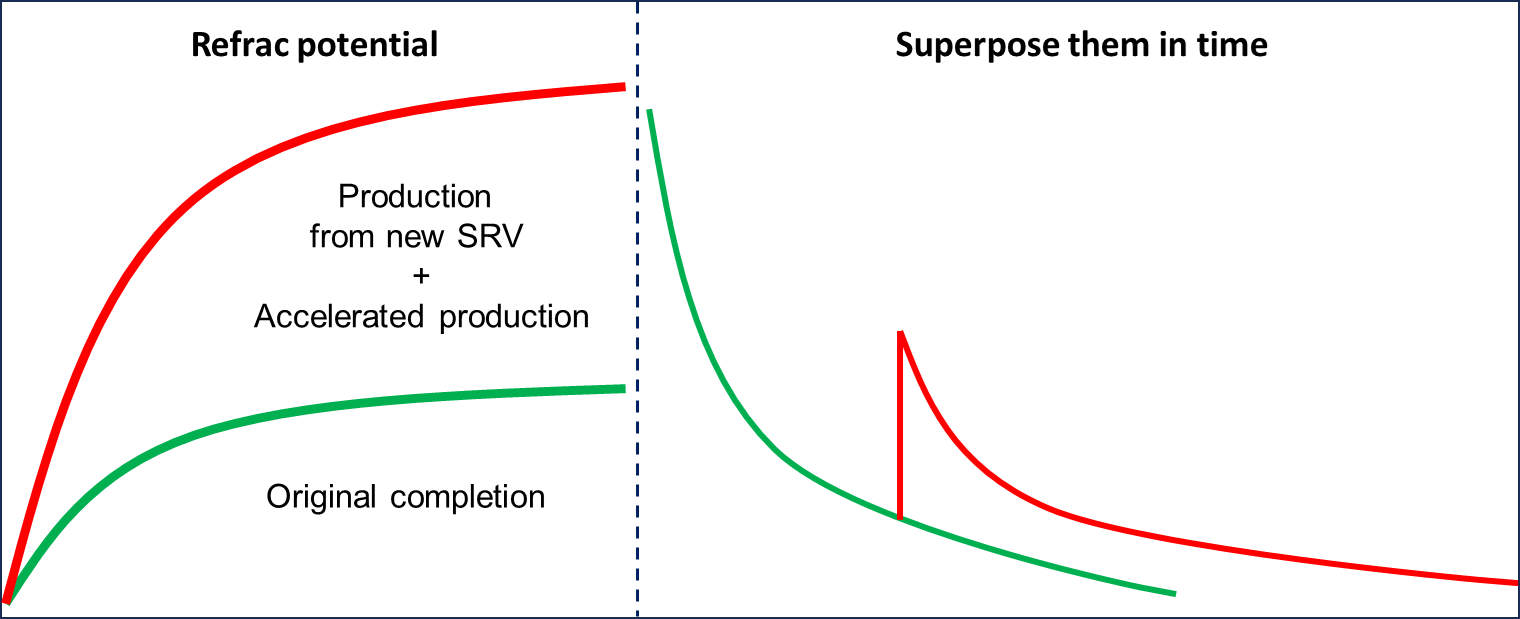Fig. 9: Forecasting the updated model and evaluating uplift

## 6. Export Dataset and Results

To date, the production forecast results can be exported in excel format in daily or monthly option, while the dataset can be exported into:

1. OPM Flow
2. Eclipse (E100)
3. CMG IMEX
4. SENSOR

Let us know, if you'd like us to add another export option for your company. Contact us or use the Send Feedback button at the top right of whitson+.